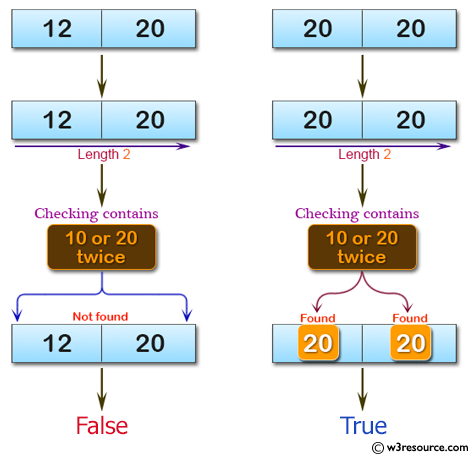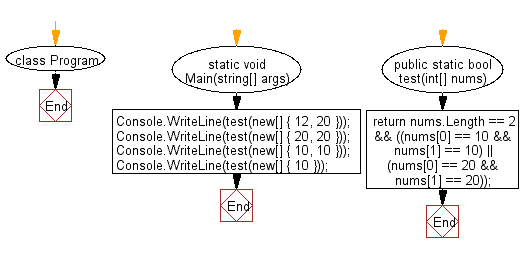﻿ C# - Return true if the array contains 10 or 20 twice# C# Sharp Basic Algorithm Exercises: Check a given array of integers and return true if the array contains 10 or 20 twice

## C# Sharp Basic Algorithm: Exercise-100 with Solution

Write a C# Sharp program to check a given array of integers and return true if the array contains 10 or 20 twice. The length of the array will be 0, 1, or 2.

Pictorial Presentation:Sample Solution:-

C# Sharp Code:

``````using System;
namespace exercises
{
class Program
{
static void Main(string[] args)
{

Console.WriteLine(test(new[] { 12, 20 }));
Console.WriteLine(test(new[] { 20, 20 }));
Console.WriteLine(test(new[] { 10, 10 }));
Console.WriteLine(test(new[] { 10 }));

}

public static bool test(int[] nums)
{
return nums.Length == 2
&& ((nums == 10 && nums == 10)
|| (nums == 20 && nums == 20));
}
}
}
```
```

Sample Output:

```False
True
True
False
```

Flowchart:C# Sharp Code Editor:

Improve this sample solution and post your code through Disqus

What is the difficulty level of this exercise?

Test your Programming skills with w3resource's quiz.

﻿